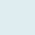# Learn with

Understand about MS Excel with more than 150 questions covering multiple topics. After taking Quizzes, you will be redirected to the correct answer, where you will find an explanation of each question at length.

Don’t forget to Bookmark this Quiz page to come back later.Before you start:

• 160+ questions are spread into 13 sets, and each set has 8-15 questions.
• Every question has only one correct answer.
• No negative marking
• After finishing a set of quizzes, you will see the number of the correct answer for a few seconds.
• Lastly, redirection to correct answers

## General Introduction

In the Introduction to Excel Quiz, you will find 15 questions and later find answers as well. The questions will familiarize you with an introductory overview of Excel.

## Entering and Editing

Quiz on Entering and Editing Excel Worksheet with 10 questions. This will cover entering and managing data in your worksheet like Number, Text, Date, or Time.

## Worksheet Operation

10 questions on basic understanding of ways of an Excel workbook and worksheet operates. Discovering tips and techniques for managing and controlling your sheets.

## Formatting

Understand formatting and styling in an Excel Worksheet with 10 questions. A simple understanding of formatting will make your Excel worksheet stylish that may help to avoid errors.

## Files and Template

Learn managing Excel Files and Templates with 8 questions like saving or setting a password that is performing actions with workbook files.

## Range and Table

12 questions to understand editing and manipulating Excel cells by implementing Tables and Ranges in Excel.

## Printing

Learn with 9 quizzes about Print on an Excel workbook or a specific range of data following the most recommended steps to avoid any mistakes.

## Formula and Functions (Basics - 1)

Part 1– Learn about Excel Formula and Function basics with 15 questions. Basics of using Excel built-in functions for calculations.

## Formula and Functions (Basics - 2)

Part 2– Learn about Excel Formula and Function basics with 15 questions. Know more about predefined Excel functions with Trivia.

## Excel Shortcut (Part - 1)

Part 1 of the Excel shortcut quiz (15 Trivia). You will learn shortcuts in two parts; before that, try to answer quizzes and later find answers.

## Excel Shortcut (Part - 2)

Part 2 of the Excel shortcut quiz (15 Trivia), a step ahead. To test your grip and understand more about Excel Shortcuts.

## Formula and Functions (Beyond Basics - 1)

Part 1 – Check out quizzes related to Excel formula and function going beyond basics 🧮. Learn how functions are implemented in formula (15 quizzes).

## Formula and Functions (Beyond Basics - 2)

Part 2 – Learn about Excel formula and function with a Trivia 🧮 and later you will find answers. Learn how functions are implemented in formula (15 quizzes)